# Algebra 1 : Decimals and Percentage

## Example Questions

### Example Question #71 : Decimals And Percentage

John makes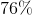of the shots he takes in a basketball game. What is this number written as a decimal?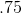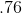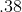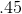Explanation:

To find a decimal from a percent, you just need to put the percent overSo: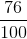This is equivalent to seventy-six hundreths which, as a decimal is written as.

### Example Question #3001 : Algebra 1

If you owe the bank% of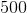dollars, how much money do you owe them?  Round your answer to the nearest cent.Explanation:

To solve this problem, first, you must convert the percentage above into a decimal.  To find the decimal equivalent of a percentage, simply move the decimal (if there is none, remember that there is always an implied decimal after the one's digit) to the left twice.  Then, you take your result and multiply it by the initial amount for your answer: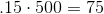### Example Question #71 : Decimals And Percentage

Which of the following is the decimal equivalent of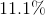?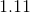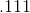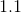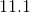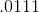Explanation:

To find the decimal equivalent of a percentage, simply move the decimal (if there is none, remember that there is always an implied decimal after the one's digit) to the left twice.  The best answer is:### Example Question #71 : Decimals And Percentage

Which of the following is the decimal equivalent of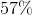?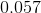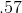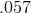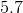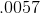Explanation:

To find the decimal equivalent of a percentage, simply move the decimal (if there is none, remember that there is always an implied decimal after the one's digit) to the left twice.  The best answer is:### Example Question #3002 : Algebra 1

If you owe the bank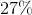ofdollars, how much money do you owe them?  Round your answer to the nearest cent.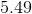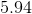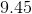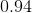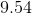Explanation:

To solve this problem, first, you must convert the percentage above into a decimal.  To find the decimal equivalent of a percentage, simply move the decimal (if there is none, remember that there is always an implied decimal after the one's digit) to the left twice.  Then, you take your result and multiply it by the initial amount for your answer: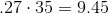### Example Question #3001 : Algebra 1

Which of the following is the decimal equivalent of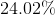?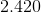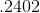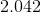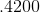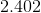Explanation:

To find the decimal equivalent of a percentage, simply move the decimal (if there is none, remember that there is always an implied decimal after the one's digit) to the left twice.  The best answer is:### Example Question #77 : Decimals And Percentage

Which of the following is the decimal equivalent of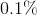?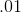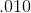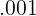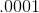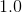Explanation:

To find the decimal equivalent of a percentage, simply move the decimal (if there is none, remember that there is always an implied decimal after the one's digit) to the left twice.  The best answer is:### Example Question #3001 : Algebra 1

If I still owe my friend Ralphof thedollars he loaned me, how much money do I still owe Ralph?  Round your answer to the nearest cent.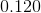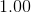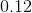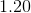Explanation:

To solve this problem, first, you must convert the percentage above into a decimal.  To find the decimal equivalent of a percentage, simply move the decimal (if there is none, remember that there is always an implied decimal after the one's digit) to the left twice.  Then, you take your result and multiply it by the initial amount for your answer:### Example Question #3001 : Algebra 1

If you owe the bankof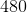dollars, how much money do you owe them?  Round your answer to the nearest cent.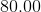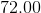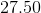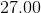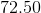Explanation:

To solve this problem, first, you must convert the percentage above into a decimal.  To find the decimal equivalent of a percentage, simply move the decimal (if there is none, remember that there is always an implied decimal after the one's digit) to the left twice.  Then, you take your result and multiply it by the initial amount for your answer:### Example Question #71 : Decimals And Percentage

If 37% of a pie was consumed, what is the decimal equivalence to how much of the pie is missing?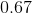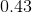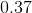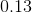Explanation:

This problem is simply asking us to convert 37% into its decimal equivalence.

The quickest way to switch between decimals and percentage is by keeping in mind that it is a factor of 100. For instance, decimals may be converted to percents by multiplying by 100 whereas turning percents to decimals would be the opposite operation (division). This is true because by definition, a percentage is a portion of 100. In this case, we are interested in a portion of 37.

Given this information,

we may convert from percent to decimal. This would require dividing by 100.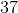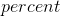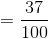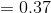### All Algebra 1 Resources